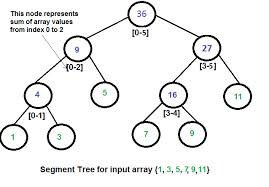# Help on range updates in Segment Tree

Can anyone please tell me how to update an interval in segment tree?

1 Like

Actually I Think you misunderstood the concept of segment tree as your heading refers to another data structure ( Interval Tree ) but your question is on Segment tree.

Interval Tree and Segment tree are two different ( but Similar ) Data structures.

I assume that you are asking to explain the update function in segment tree.

Segment trees are used to answer range queries efficiently. For example let us consider Range Sum query.Now that I assume that you know how to build a tree , so if i want to update the value of 1st element then i have to update the tree in such a way that all the nodes which cover the first element must be updated.That can be done with the following function. here a and b are the limits and i is the index where you r updating so if i is in the range of a and b ==> if a <= i <= b then we must update the node else no need to do anything.

``````void update_sum(long int n ,long  int a ,long  int b, long int i ,int value){

if( a > b || a > i || b < i) return;
if ( a == b ) { tree[n] = tree[n]+value; return;}

update_sum( 2*n, a , (a+b)/2 , i, value);
update_sum( 2*n+1, (a+b)/2 + 1 , b , i , value);

tree[n] = tree[2*n] + tree[2*n + 1];

}``````
2 Likes

actually i want to ask that if we have to update not on a particular index but in a range then how will it be done?

If anyone could provide a good and detailed code for this, it would be really, really usefulThe above link seems a good place, but, I still can’t understand very well myself…

Best,

Bruno

PS: I’ve changed the question title to make it more understandable.

1 Like

I want to solve it using segment tree instead of BIT as i don’t know anything about BIT…

I’m also still learning about Segment Tree/BIT and from what I understood, they are more or less equivalent, but, as Segtrees are more generally used, I think learning them would be better indeed.
Do you recommend some problems for a beginner?

@kuruma ckeck out this thread for problems on segtrees/BIT

there are also problems classified on lightOJ…
http://www.lightoj.com/volume_problemcategory.php?user_id=13603&main_category=Data%20Structures

1 Like

@infinitum How can this be done with lazy propogation?